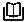# 数组表达式

#### IN

expression IN (value [, ...])

```SELECT 8000+500 IN (10000, 9000) AS RESULT;
result
----------
f
(1 row)```说明：

#### NOT IN

expression NOT IN (value [, ...])

```SELECT 8000+500 NOT IN (10000, 9000) AS RESULT;
result
----------
t
(1 row)```说明：

#### ANY/SOME (array)

expression operator ANY (array expression)

expression operator SOME (array expression)

```SELECT 8000+500 < SOME (array[10000,9000]) AS RESULT;
result
----------
t
(1 row)
```
```SELECT 8000+500 < ANY (array[10000,9000]) AS RESULT;
result
----------
t
(1 row)```

• 如果对比结果至少获取一个真值，则ANY的结果为true。
• 如果对比结果没有真值，则ANY的结果为false。说明：

SOME是ANY的同义词。

#### ALL (array)

expression operator ALL (array expression)

• 如果所有的比较结果都为真值（包括数组不含任何元素的情况），则ALL的结果为true。
• 如果结果有任一结果是false，则ALL的结果为false。

```SELECT 8000+500 < ALL (array[10000,9000]) AS RESULT;
result
----------
t
(1 row)```

# 相关产品

### 提交成功！

*必选